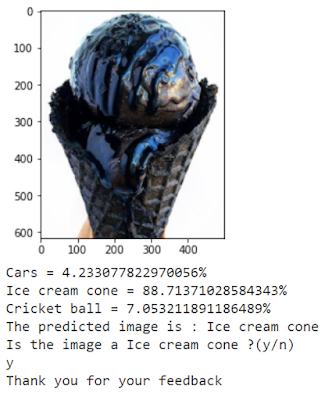# SVM Algorithm Steps and Image Classification With Support Vector Machine (SVM)

SVM Algorithm Steps and Image Classification With Support Vector Machine (SVM) – What is SVMIt’s been a long time since I’ve written an article that specifically discusses how an algorithm works. This time, mimin will discuss one of the algorithms that are often used, namely SVM!

At the end of the article, there will be a brief discussion of SVM for image classification.

Come on, let’s start the article!

What is SVM? Support Vector Machine (SVM) is a machine learning algorithm that is supervised. This algorithm is used to solve classification and regression problems.

Because it is useful for classification, this SVM will divide data points into certain groups. However, what are the ‘dividers’ or ‘separators’ between these groups?

Well, to classify data points into groups, SVM has a feature called “hyperplane

For example, you can see in the image below, where the hyperplane is the dividing line between the green and red groups.

Then how to determine where the hyperplane is placed?

In SVM, the hyperplane will be placed at the midpoint of the outermost data point of each group. For convenience, we assume that there are only 2 features that are used, x and y. The distance between the outermost point and the hyperplane is called the margin, guys.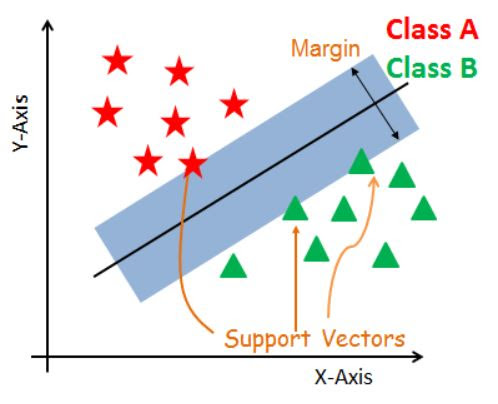We call this hyperplane determination as Maximal Margin Classifier, because this hyperplane produces the widest margin for data points in both groups (Class A and Class B).

To understand better, imagine if the hyperplane is shifted to the left, the margin will be bigger for Class B compared to Class A. So Class B has the widest margin.

So far, it still looks simple, because the data has been divided into 2 completely separate groups. But in reality, the data we have will not be “clean” that way.

What if the case is like the example picture on the left? If you keep using the Maximal Margin Classifier, the result will be like the picture on the right.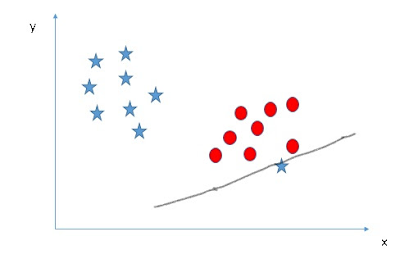The classification results are ridiculous. From here, we can tell that the Maximal Margin Classifier is very sensitive to outliers. To prevent this from happening, we can use the Soft Margin Classifier, where SVM is allowed to ignore outliers, so there is no misclassification of data.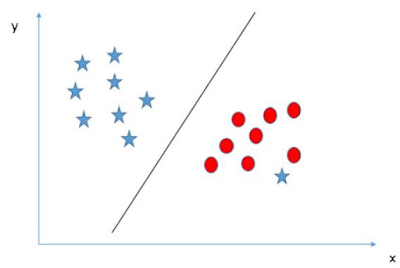Back to reality, there are very few outliers in the dataset, only 1. So what if there are many outliers? Is the amount of data that can be misclassified = the number of outliers?

To find out how much misclassification is allowed into the Soft Margin, we have to use Cross Validation.

Hopefully it’s still digestible up to here. Now, let’s discuss for more complex cases. For example, do you have data like this, how do you do it?

how to determine the hyperplane?

Since it is not possible to draw a straight line that can divide the data into 2 groups, then we can add an additional feature, namely z! Mathematically, we can get feature z by calculating z=x^2+y^2. If we find z, we can plot the data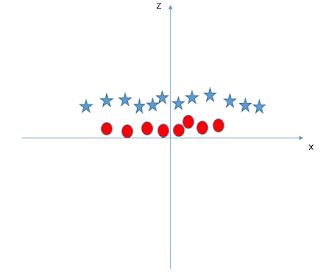This way, the differences between the 2 groups will be clearer and you can create a hyperplane.

This technique of adding new features is known as the kernel trick (a method that transforms a low dimensional input space into a larger dimension). Kernel trick can be used to separate inseparable data like this.

Now, we can create a hyperplane with the addition of feature z earlier, so if we look at the initial conditions, aka there are only features x and y, the hyperplane looks like this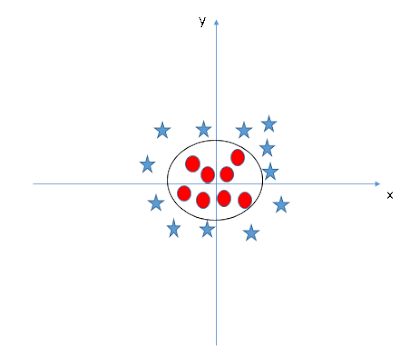That’s it guys at a glance how SVM works. You can use this method to classify, whether it’s images, text, or other classifications. In this article, we will discuss the application of SVM specifically for image classification, okay?

The process is as follows guys:

(1). Provide input

The main task of SVM in this case is to classify the images that we provide. Because SVM is a supervised model, the input that we provide already has a label.

For example, you want to ask SVM to guess the picture of a car, ice cream, or ball. Now, we input the three images that have been given information, for example the car image is labeled 0, ice cream 1, and ball 2.

Oh yes, this SVM reads images not in the form of images, guys! But in the form of a set of pixels like the image below.

If you notice, the set of pixels contains the right numbers. Usually, the value of this number ranges from 0 (black) – 255 (white).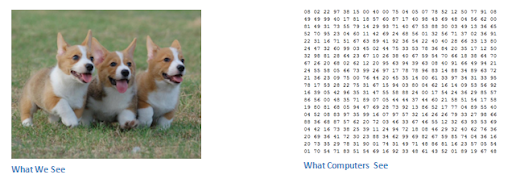For the size itself, the size is width x height x RGB channel values. If you do image classification using SVM, don’t forget to match all the sizes of your input images, because SVM cannot process images of various sizes.

(2). Split input

The step that we should not forget is to separate the dataset into training and testing. Training data is used to train the model while testing data will be used to test whether the model can work well.

(3). Modeling

If you have determined the data for training, then all you have to do is input the code to create the SVM model and use the training data to train the model.

(4). Model evaluation

As Mimin said, we will test again whether the model is okay or not. Well, we can see the accuracy of the model when tested using the testing data earlier.

If the model is OK, then we can use it to do image classification. Here’s an example of the output, guys: